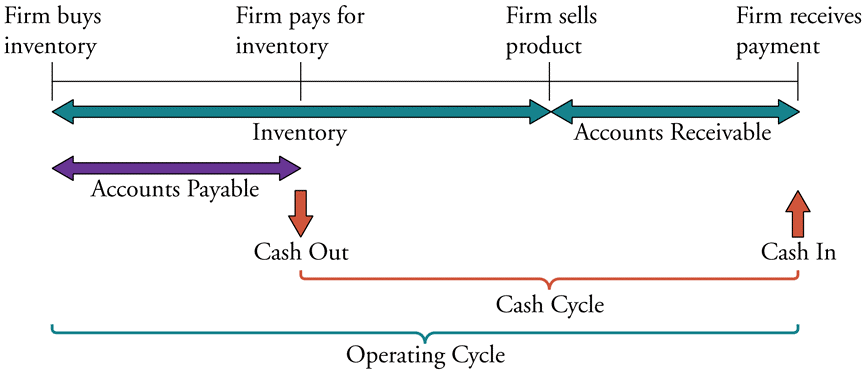# Fight Finance

#### CoursesTagsRandomAllRecentScores

Which of the following statements is NOT correct?

High risk firms in danger of bankruptcy tend to have:

Question 472  quick ratio, accounting ratio

A firm has current assets totaling $1.5b of which cash is$0.25b and inventories is $0.5b. Current liabilities total$2b of which accounts payable is $1b. What is the firm's quick ratio, also known as the acid test ratio? Which of the following is NOT a synonym of 'required return'? High risk firms in danger of bankruptcy tend to have: A firm has a debt-to-equity ratio of 25%. What is its debt-to-assets ratio? A firm has a debt-to-equity ratio of 60%. What is its debt-to-assets ratio? A firm has a debt-to-assets ratio of 20%. What is its debt-to-equity ratio? In the home loan market, the acronym LVR stands for Loan to Valuation Ratio. If you bought a house worth one million dollars, partly funded by an$800,000 home loan, then your LVR was 80%. The LVR is equivalent to which of the following ratios?

Safe firms with low chances of bankruptcy will tend to have:

The below diagram shows a firm’s cash cycle.Which of the following statements about companies’ cash cycle is NOT correct?

The Du Pont formula is:

$$\dfrac{\text{Net Profit}}{\text{Sales}} \times \dfrac{\text{Sales}}{\text{Total Assets}} \times \dfrac{\text{Total Assets}}{\text{Owners' Equity}}$$

Which of the following statements about the Du Pont formula is NOT correct?

A company manager is thinking about the firm's book assets-to-equity ratio, also called the 'equity multiplier' in the Du Pont formula:

$$\text{Equity multiplier} = \dfrac{\text{Total Assets}}{\text{Owners' Equity}}$$

What's the name of the decision that the manager is thinking about? In other words, the assets-to-equity ratio is the main subject of what decision?

Note: Du Pont formula for analysing book return on equity:

\begin{aligned} \text{ROE} &= \dfrac{\text{Net Profit}}{\text{Sales}} \times \dfrac{\text{Sales}}{\text{Total Assets}} \times \dfrac{\text{Total Assets}}{\text{Owners' Equity}} \\ &= \text{Net profit margin} \times \text{Total asset turnover} \times \text{Equity multiplier} \\ \end{aligned}